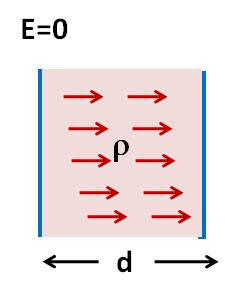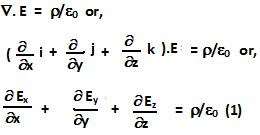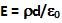## Monday, July 5, 2010

### Irodov Problem 3.52Let the left plate be at a higher potential than the right plate. So the direction of the electric field will be from left plate to right plate. Further let us suppose that the Electric field at the left plate is equal to zero. We know that,Since the parallel plates are large, the direction of electric field will be along the x-direction (from the left plate to the right one). This means that,Hence, we can determine the electric field as a function of distance form the left plate as,At the right plate x = d, and and so the electric field at the right plate is,We also know that,